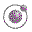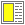Expressions, Types, and Functions - slide 24 : 46

Tables as lists of rows

It is natural to represent tables as lists of rows, and to represent a row as a list

Tables play an important roles in many web documents

LAML has a strong support of tables

Expression

Value

`tab1`
```(("This" "is" "first" "row")
("This" "is" "second" "row")
("This" "is" "third" "row")
("This" "is" "fourth" "row")
)```
`(show-table tab1)`
 This is first row This is second row This is third row This is fourth row
`(show-table (transpose-1 tab1))`
 This This This This is is is is first second third fourth row row row row
`(show-table (eliminate-row 2 tab1))`
 This is first row This is third row This is fourth row
`(show-table (eliminate-column 4 tab1))`
 This is first This is second This is third This is fourth
Later in this material we will study possible implementations of the function show-table